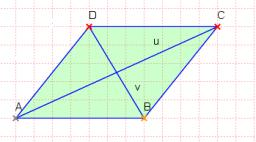# Circumference 4285

Calculate the circumference of a rhombus, one side of which is 6 cm long, and the height of this side is 2 cm long. The height on the other side is 4 cm long.

x =  0 cm

### Step-by-step explanation:Did you find an error or inaccuracy? Feel free to write us. Thank you!

Tips for related online calculators
Do you have a linear equation or system of equations and looking for its solution? Or do you have a quadratic equation?

#### Grade of the word problem:

We encourage you to watch this tutorial video on this math problem: